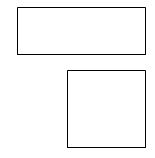# Word Problems and Finding Numbers Halfway Between Two Others

Word Problems

Many top schools and the 11+ system will be keen to test your child's maths abilities separately from their English skills. However, the expectation is there that children will be able to solve problems presented in word form; this discriminates against those for whom English is a foreign language but is used as an indicator of immediate ability rather than potential ability.

Questions need to be tackled systematically and the order of mathematical processes addressed before anything gets calculated. Does your child know exactly what is wanted? Have they taken the correct numbers from the question and followed the logical process to get to an answer? Have they answered the question that is being asked rather than the question that they think is being asked?

Let's look at a typical word problem and dissect it as I would expect a competent child to do at this age.

There are fifteen rows of cars in a car park. There are six rows of twenty spaces and the remainder have twelve spaces. If three quarters of the car park is full, how many spaces are there?

This is a multi-step problem as there are several parts to sort through and the answer will take a lot of thought. First, it may help to sketch the car park and jot down relevant numbers. Visualising is a key skill and helps most children a lot. Treat the two parts of the car park separately and you'll have a sketch like this:6 rows of 20 (6 x 20) = 120

15 - 6 = 9 rows of 12 = 108

Total = 120 + 108 = 228

Now you could find three quarters of 228.

'Divide by the bottom, times by the top' is the way to find a fraction of a number. We've already worked out that there are 228 spaces, so the sum is

228 ÷ 4 = 57      then     57 x 3 = 171

However, this tells us the number of cars in the car park. It's NOT the answer to the question, which asked how many SPACES there were!

The answer will be 228 - 171 = 57.

This could also have been found by cutting the step of finding three quarters of the number and simply finding one quarter in the first place.

Finding Numbers Halfway Between Two Others

This is an old chestnut and it still surprises me how often it catches out the more able pupils. The halfway point between two given digits is found by adding them together and halving the result.

For example, the number that lies halfway between 8 and 24 is found by adding 8 to 24 and dividing the answer by two.

8 + 24 = 32; 32 ÷ 2 = 16.

However, the likelihood is that your child will be asked to find the more complex answers which appear when decimals or fractions are used.

What is the number halfway between 0.8 and 1.7?

Add the two together and divide by two:
0.8 + 1.7 = 2.5; 2.5 ÷ 2 = 1.25

The fraction example would be more complex still as it would need similar denominators to add the fractions together. There is a worked example of how to add fractions.

What is the number halfway between 3 1⁄3 and 4 2⁄9?

Adding together the fractions, once we have changed the denominators to the same figure, gives seven and five ninths. This is not easy to work with as neither part can be halved so change it into an improper or top-heavy fraction. The equivalent is 68⁄9 so half of this is 34⁄9, or 37⁄9.

Finally, the use of negative numbers could throw some children.

What is the halfway point of 2 and -7?

Add together the two numbers and halve them, but remember when you add a negative number it is the same as taking the number away. 2 + ( -7 ) = -5; -5 ÷ 2 = -2.5